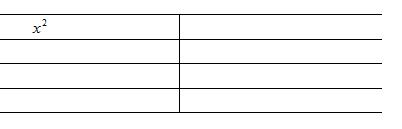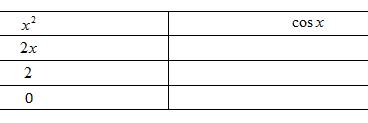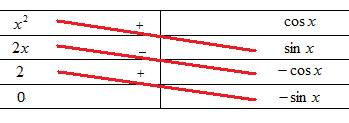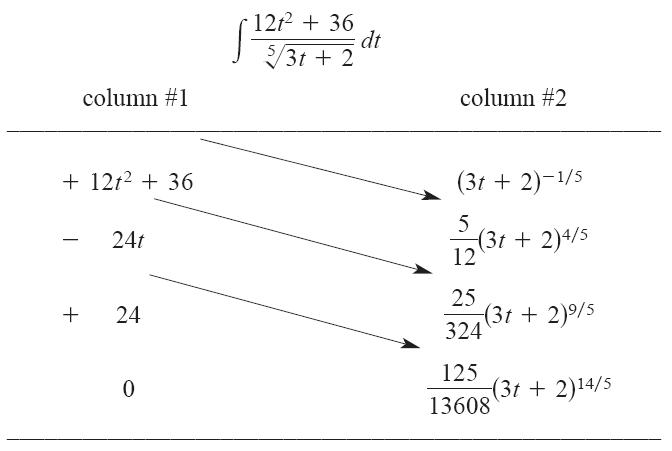# Quod Erat Demonstrandum

## 2012/02/07

### Integration by parts

Filed under: NSS,Pure Mathematics,Teaching — johnmayhk @ 9:29 上午$\int u(x)v(x)dx$

（其中$u(x)$ 是多項式，$v(x)$ 是容易計算積分的函數）

Evaluate$\int x^2\cos xdx$.$\int x^2\cos xdx=x^2\sin x-2x(-\cos x)+2(-\sin x)+C=x^2\sin x+2x\cos x-2\sin x+C$$\int \frac{12t^2+36}{\sqrt{3t+2}}dt=(5t^2+15)(3t+2)^{\frac{4}{5}}-\frac{50t}{27}(3t+2)^{\frac{9}{5}}+\frac{125}{567}(3t+2)^{\frac{14}{5}}+C$

https://www.maa.org/sites/default/files/pdf/mathdl/CMJ/Horowitz307-311.pdf

## 3 則迴響 »

1.Furthermore,
http://www.maa.org/pubs/Calc_articles/ma036.pdf

迴響 由 johnmayhk — 2012/02/07 @ 10:48 下午 | 回應

2.呢個方法幾得意^^
不過想請問下…呢個方法叫咩名 and DSE考試比唔比用呢個方法??

迴響 由 sze long — 2012/04/21 @ 4:18 下午 | 回應